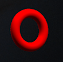Discussion about math, puzzles, games and fun.   Useful symbols: ÷ × ½ √ ∞ ≠ ≤ ≥ ≈ ⇒ ± ∈ Δ θ ∴ ∑ ∫  π  -¹ ² ³ °

You are not logged in.

## #1 2013-12-06 17:00:12

dford
Member
Registered: 2013-12-06
Posts: 4

### ABCD x E Puzzle - Solution ?

Hi this is my first post here.

On the puzzle ABCD × E = DCBA

Should it not be, (A)(B)(C)(D) x E = (D)(C)(B)(A)

There for A, B, C, D can be anything as long as E = 1 or 0?

If this has been discussed before sorry.

Thanks all!

Last edited by dford (2013-12-06 17:04:33)

Offline

## #2 2013-12-06 18:54:42

roniman
MemberRegistered: 2013-11-24
Posts: 30

### Re: ABCD x E Puzzle - Solution ?

(A)(B) is like (2)(4), which would mean 2x4.

AB is like 24

So if A =2, B = , C = , D = 8, and E = 4, then
2178
x     4
--------
8712

"Who Dares Wins" - Motto of the British Special Air Service

Offline

## #3 2013-12-06 22:56:54

bobbym
bumpkinFrom: Bumpkinland
Registered: 2009-04-12
Posts: 109,606

### Re: ABCD x E Puzzle - Solution ?

Hi;

Another solution is 1089 * 9 = 9801 provided there is no condition that all the numbers have to be different.

In mathematics, you don't understand things. You just get used to them.
If it ain't broke, fix it until it is.
Always satisfy the Prime Directive of getting the right answer above all else.

Offline

## #4 2017-07-17 09:10:19

ProgrammerCam
Member
Registered: 2017-07-17
Posts: 2

### Re: ABCD x E Puzzle - Solution ?

// ABCD * E = DCBA
#include <iostream>

using namespace std;

int main(){
int a = 0;
int b = 0;
int c = 0;
int d = 0;
int e = 0;
for(int i = 0; i < 100000; i++){
if((a!=b) && (a!=c) && (a!=d) && (a!=e) && (b!=c) && (b!=d) && (b!=e) && (c!=d) && (c!=e) && (d!=e)){
if((((a*1000) + (b*100) + (c*10) + (d*1)) * e) == ((d*1000) + (c*100) + (b*10) + (a*1))){
cout << a << b << c << d << " * " << e << " = " << d << c << b << a << endl;
}
}
if(e < 9){
e++;
}else if((e == 9) && (d < 9)){
d++;
e = 0;
}else if((e == 9) && (d == 9) && (c< 9)){
c++;
d = 0;
e = 0;
}else if((e == 9) && (d == 9) && (c == 9) && (b < 9)){
b++;
c = 0;
d = 0;
e = 0;
}
else if((e == 9) && (d == 9) && (c == 9) && (b == 9) && (a < 9)){
a++;
b = 0;
c = 0;
d = 0;
e = 0;
}else if(a == 9){
a = 0;
b = 0;
c = 0;
d = 0;
e = 0;
}
}
cout << "End";
}

----------------------------------------------------------------------------------------------------------------------------------------------------------------------------

This program in c++ will solve it.
to find out the solutions if you ignore duplicates use this:

----------------------------------------------------------------------------------------------------------------------------------------------------------------------------
// ABCD * E = DCBA
#include <iostream>

using namespace std;

int main(){
int a = 0;
int b = 0;
int c = 0;
int d = 0;
int e = 0;
for(int i = 0; i < 100000; i++){
if((((a*1000) + (b*100) + (c*10) + (d*1)) * e) == ((d*1000) + (c*100) + (b*10) + (a*1))){
cout << a << b << c << d << " * " << e << " = " << d << c << b << a << endl;
}
if(e < 9){
e++;
}else if((e == 9) && (d < 9)){
d++;
e = 0;
}else if((e == 9) && (d == 9) && (c< 9)){
c++;
d = 0;
e = 0;
}else if((e == 9) && (d == 9) && (c == 9) && (b < 9)){
b++;
c = 0;
d = 0;
e = 0;
}
else if((e == 9) && (d == 9) && (c == 9) && (b == 9) && (a < 9)){
a++;
b = 0;
c = 0;
d = 0;
e = 0;
}else if(a == 9){
a = 0;
b = 0;
c = 0;
d = 0;
e = 0;
}
}
cout << "End";
}

Offline

## #5 2017-07-17 15:03:30

Agnishom
Real MemberFrom: Riemann Sphere
Registered: 2011-01-29
Posts: 24,861
Website

### Re: ABCD x E Puzzle - Solution ?

Hi;

That's a large program. What do you do when there are 30 variables?

'And fun? If maths is fun, then getting a tooth extraction is fun. A viral infection is fun. Rabies shots are fun.'
'God exists because Mathematics is consistent, and the devil exists because we cannot prove it'
I'm not crazy, my mother had me tested.

Offline